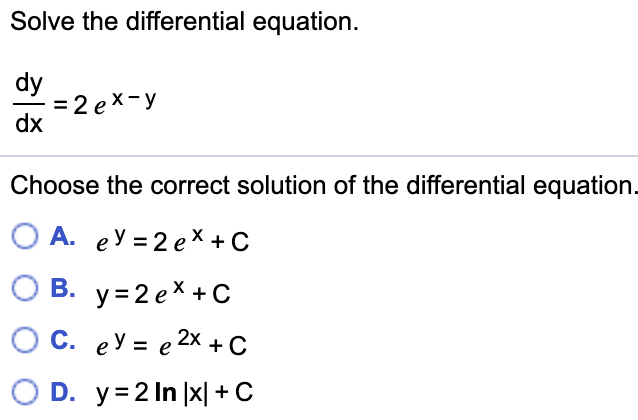# Solve the differential equation. dy 3D 2 ех-у dx Choose the correct solution of the differential equation. О А. еУ 32еХ+С О В. у32ех +C С. еУ %3D е 2х + с O D. y 2 In x| C

Question

How do I show the correct solution for the differential equation? I know the answer is D.help_outlineImage TranscriptioncloseSolve the differential equation. dy 3D 2 ех-у dx Choose the correct solution of the differential equation. О А. еУ 32еХ+С О В. у32ех +C С. еУ %3D е 2х + с O D. y 2 In x| C fullscreen